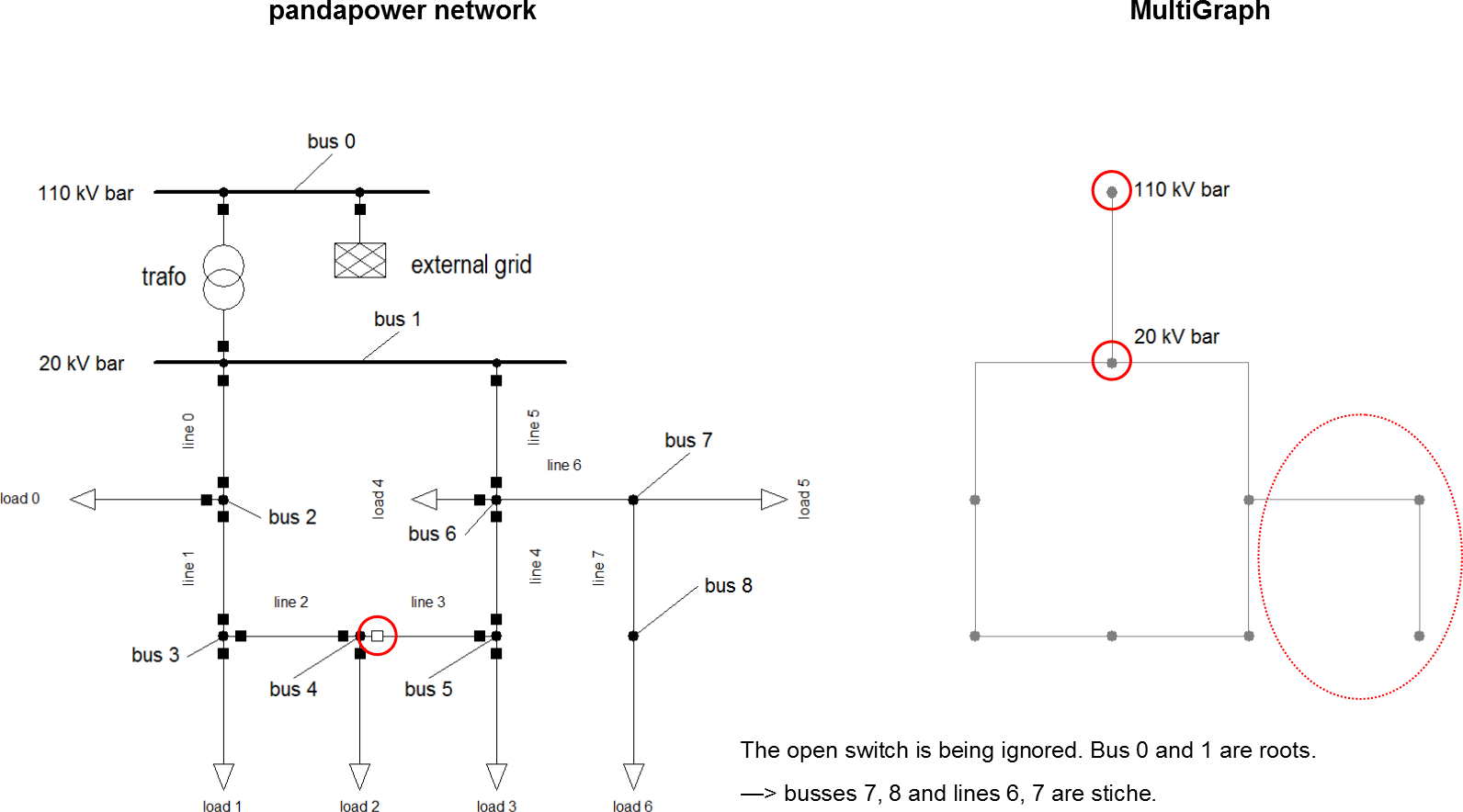# Examples

The combination of a suitable MultiGraph and the availabe topology functions enables you to perform a wide range of topological searches and analyses.

Here are a few examples of what you can do:

basic example network

```import pandapower as pp

net = pp.create_empty_network()

pp.create_bus(net, name = "110 kV bar", vn_kv = 110, type = 'b')
pp.create_bus(net, name = "20 kV bar", vn_kv = 20, type = 'b')
pp.create_bus(net, name = "bus 2", vn_kv = 20, type = 'b')
pp.create_bus(net, name = "bus 3", vn_kv = 20, type = 'b')
pp.create_bus(net, name = "bus 4", vn_kv = 20, type = 'b')
pp.create_bus(net, name = "bus 5", vn_kv = 20, type = 'b')
pp.create_bus(net, name = "bus 6", vn_kv = 20, type = 'b')

pp.create_ext_grid(net, 0, vm_pu = 1)

pp.create_line(net, name = "line 0", from_bus = 1, to_bus = 2, length_km = 1, std_type = "NAYY 4x150 SE")
pp.create_line(net, name = "line 1", from_bus = 2, to_bus = 3, length_km = 1, std_type = "NAYY 4x150 SE")
pp.create_line(net, name = "line 2", from_bus = 3, to_bus = 4, length_km = 1, std_type = "NAYY 4x150 SE")
pp.create_line(net, name = "line 3", from_bus = 4, to_bus = 5, length_km = 1, std_type = "NAYY 4x150 SE")
pp.create_line(net, name = "line 4", from_bus = 5, to_bus = 6, length_km = 1, std_type = "NAYY 4x150 SE")
pp.create_line(net, name = "line 5", from_bus = 6, to_bus = 1, length_km = 1, std_type = "NAYY 4x150 SE")

pp.create_transformer_from_parameters(net, hv_bus=0, lv_bus=1, i0_percent=0.038, pfe_kw=11.6,
vkr_percent=0.322, sn_mva=40, vn_lv_kv=22.0, vn_hv_kv=110.0, vk_percent=17.8)

pp.create_load(net, 2, p_mw = 1, q_mvar = 0.2, name = "load 0")
pp.create_load(net, 3, p_mw = 1, q_mvar = 0.2, name = "load 1")
pp.create_load(net, 4, p_mw = 1, q_mvar = 0.2, name = "load 2")
pp.create_load(net, 5, p_mw = 1, q_mvar = 0.2, name = "load 3")
pp.create_load(net, 6, p_mw = 1, q_mvar = 0.2, name = "load 4")

pp.create_switch(net, bus = 1, element = 0, et = 'l')
pp.create_switch(net, bus = 2, element = 0, et = 'l')
pp.create_switch(net, bus = 2, element = 1, et = 'l')
pp.create_switch(net, bus = 3, element = 1, et = 'l')
pp.create_switch(net, bus = 3, element = 2, et = 'l')
pp.create_switch(net, bus = 4, element = 2, et = 'l')
pp.create_switch(net, bus = 4, element = 3, et = 'l', closed = 0)
pp.create_switch(net, bus = 5, element = 3, et = 'l')
pp.create_switch(net, bus = 5, element = 4, et = 'l')
pp.create_switch(net, bus = 6, element = 4, et = 'l')
pp.create_switch(net, bus = 6, element = 5, et = 'l')
pp.create_switch(net, bus = 1, element = 5, et = 'l')
```

## Using NetworkX algorithms: shortest path

For many basic network analyses the algorithms that come with the NetworkX package will work just fine and you won’t need one of the spezialised topology functions. Finding the shortest path between two buses is a good example for that.

```import pandapower.topology as top
import networkx as nx

mg = top.create_nxgraph(net)
nx.shortest_path(mg, 0, 5)
```
```Out: [0, 1, 6, 5]
```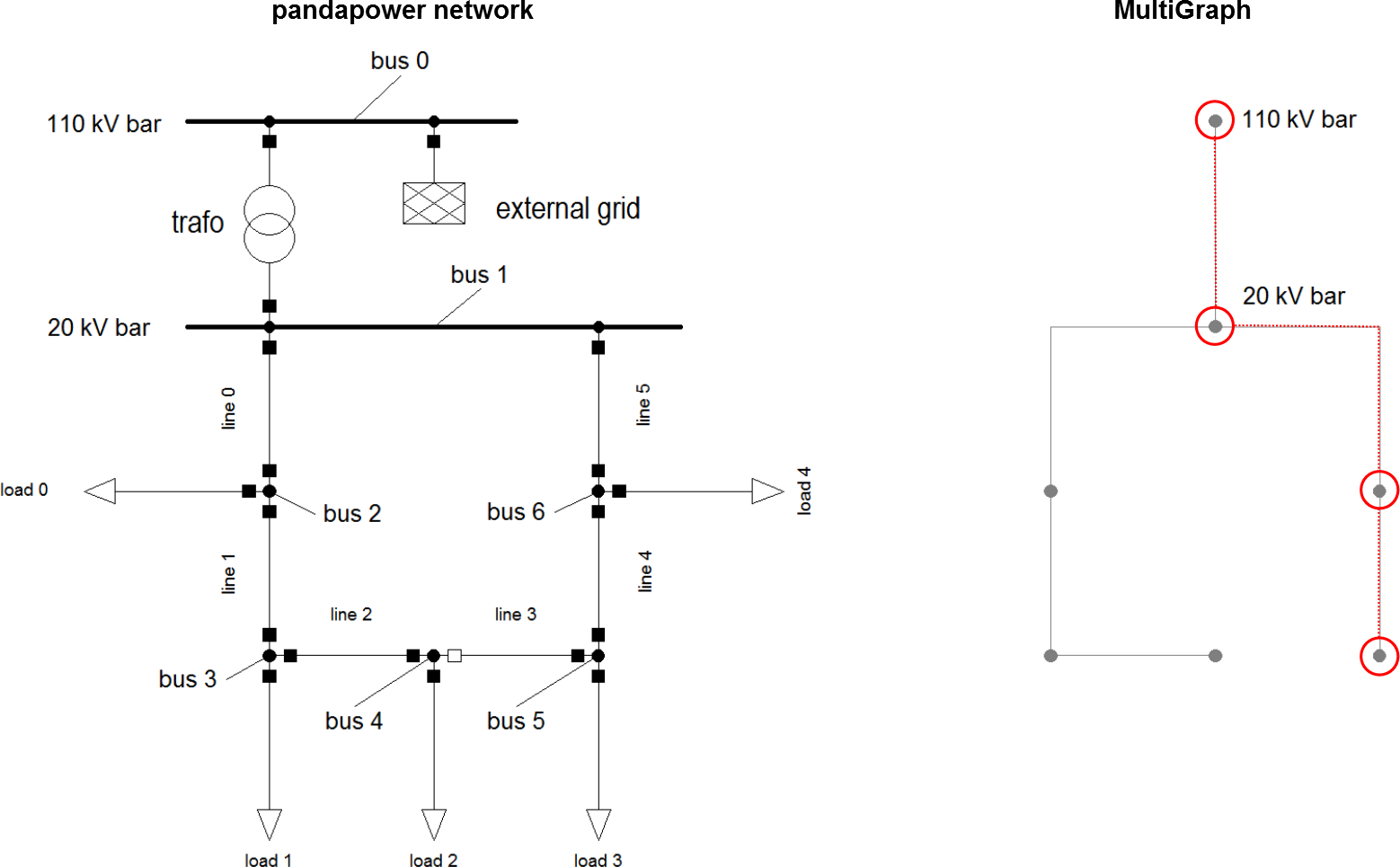## Find disconnected buses

With unsupplied_buses you can easily find buses that are not connected to an external grid.

```import pandapower.topology as top

net.switch.closed.at = 0
top.unsupplied_buses(net)
```
```Out: {5, 6}
```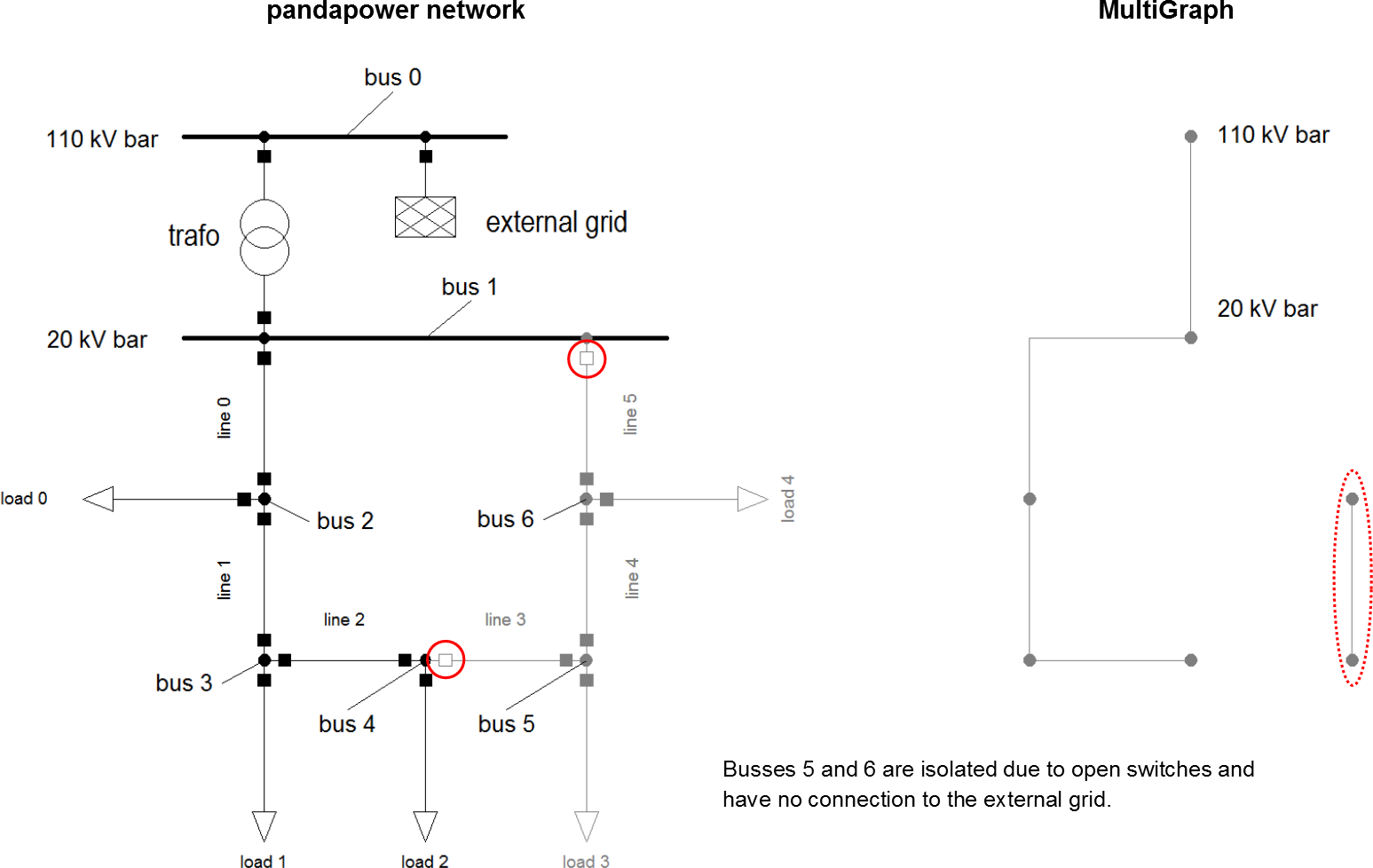## Calculate distances between buses

calc_distance_to_bus allows you to calculate the distance ( = shortest network route) from one bus all other ones. This is possible since line lengths are being transferred into the MultiGraph as an edge attribute. (Note: bus-bus-switches and trafos are interpreted as edges with length = 0)

```import pandapower.topology as top

net.switch.closed.at = 1
net.switch.closed.at = 0
top.calc_distance_to_bus(net, 1)
```
```Out:
0    0
1    0
2    1
3    2
4    3
5    4
6    1
```

Interpretation: The distance between bus 1 and itself is 0 km. Bus 1 is also 0 km away from bus 0, since they are connected with a transformer. The shortest path between bus 1 and bus 5 is 4 km long.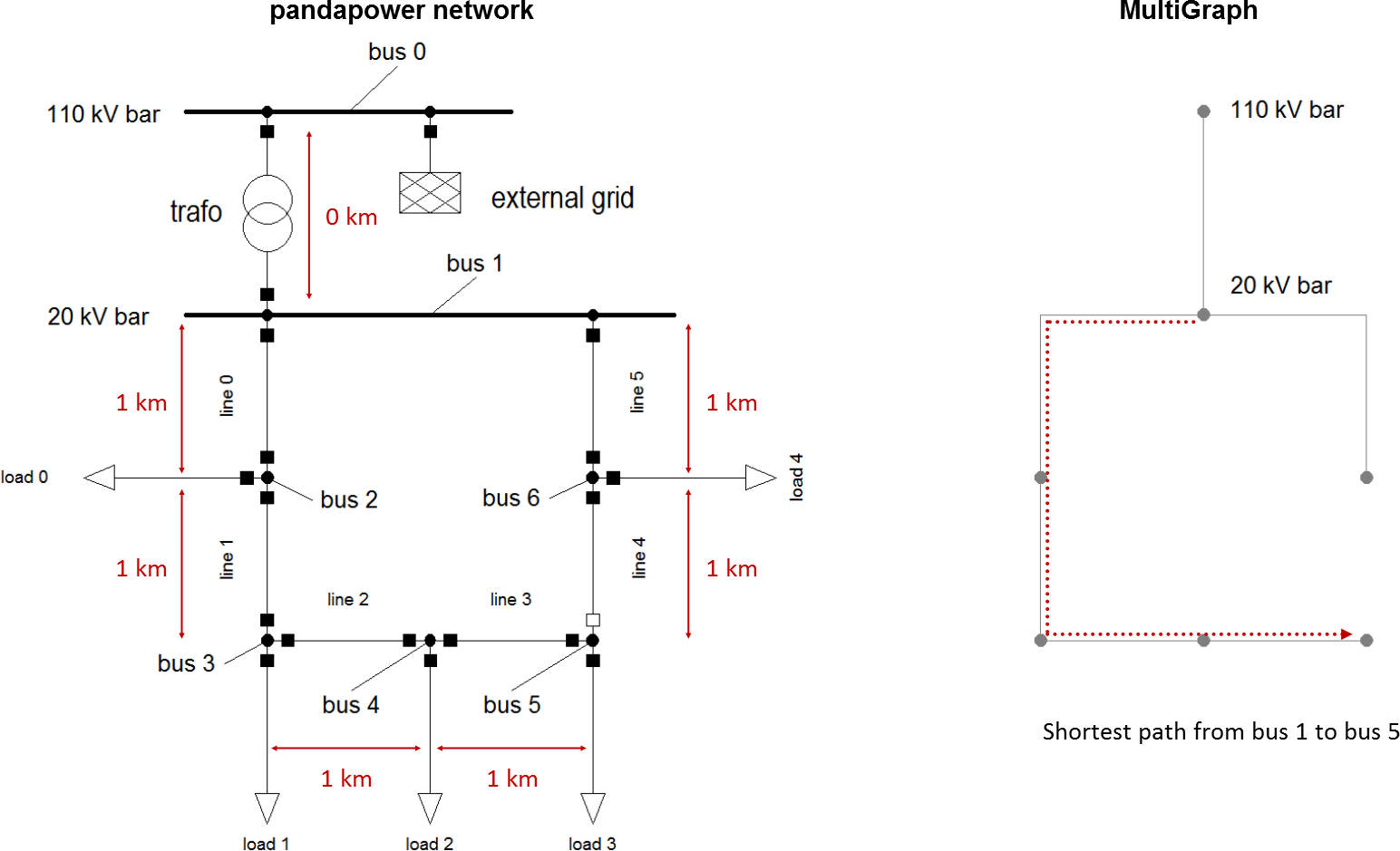## Find connected buses with the same voltage level

```import pandapower.topology as top

mg_no_trafos = top.create_nxgraph(net, include_trafos = False)
cc = top.connected_components(mg_no_trafos)
```
```In      : next(cc)
Out     : {0}
In      : next(cc)
Out     : {1, 2, 3, 4, 5, 6}
```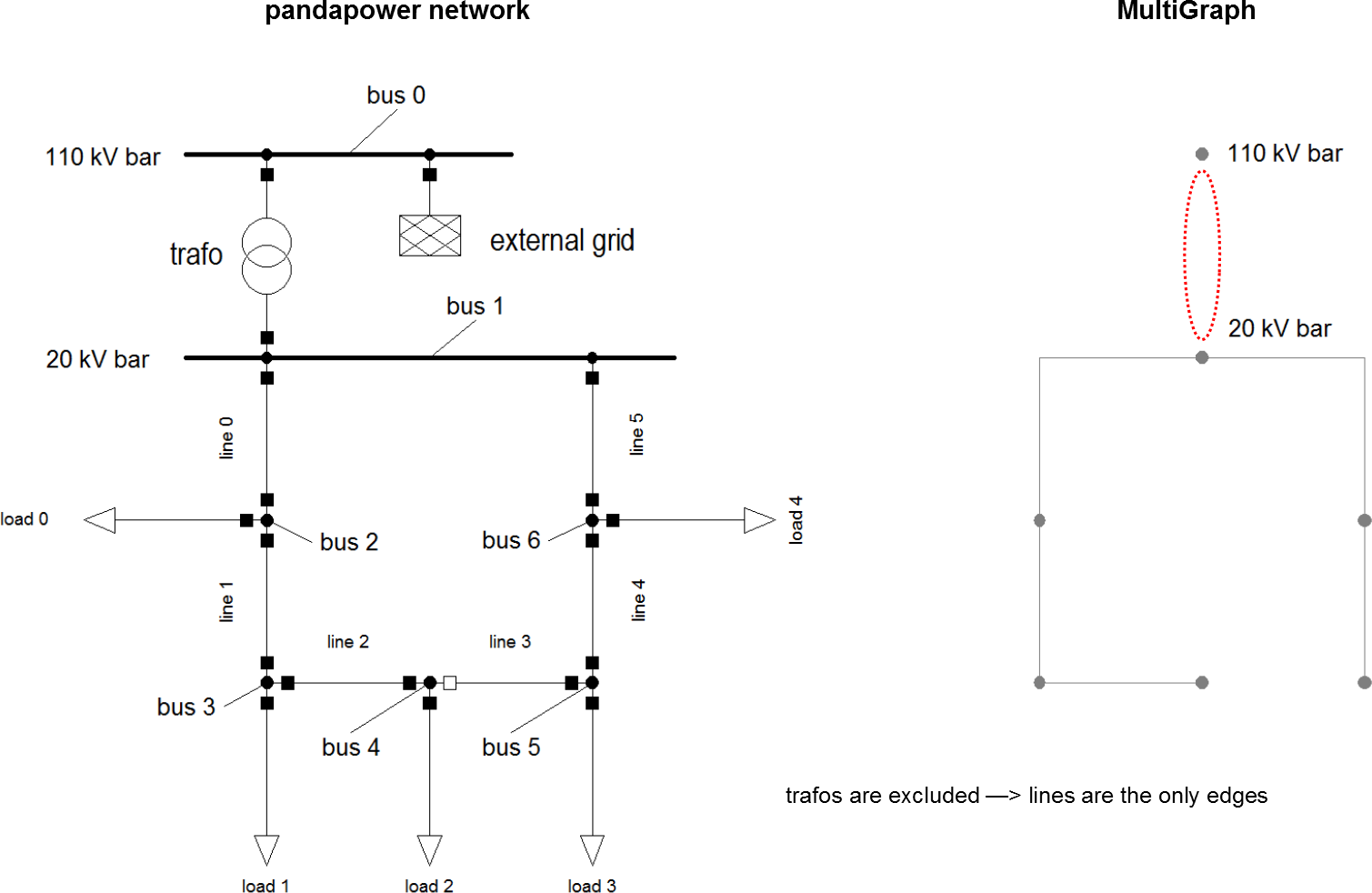## Find rings and ring sections

Another example of what you can do with the right combination of input arguments when creating the MultiGraph is finding rings and ring sections in your network. To achieve that for our example network, the trafo buses needs to be set as a nogobuses. With respect_switches = True you get the ring sections, with respect_switches = False the whole ring.

```import pandapower.topology as top

mg_ring_sections = top.create_nxgraph(net, nogobuses = [0, 1])
cc_ring_sections = top.connected_components(mg_ring_sections)
```
```In      : next(cc_ring_sections)
Out     : {2, 3, 4}

In      : next(cc_ring_sections)
Out     : {5, 6}
```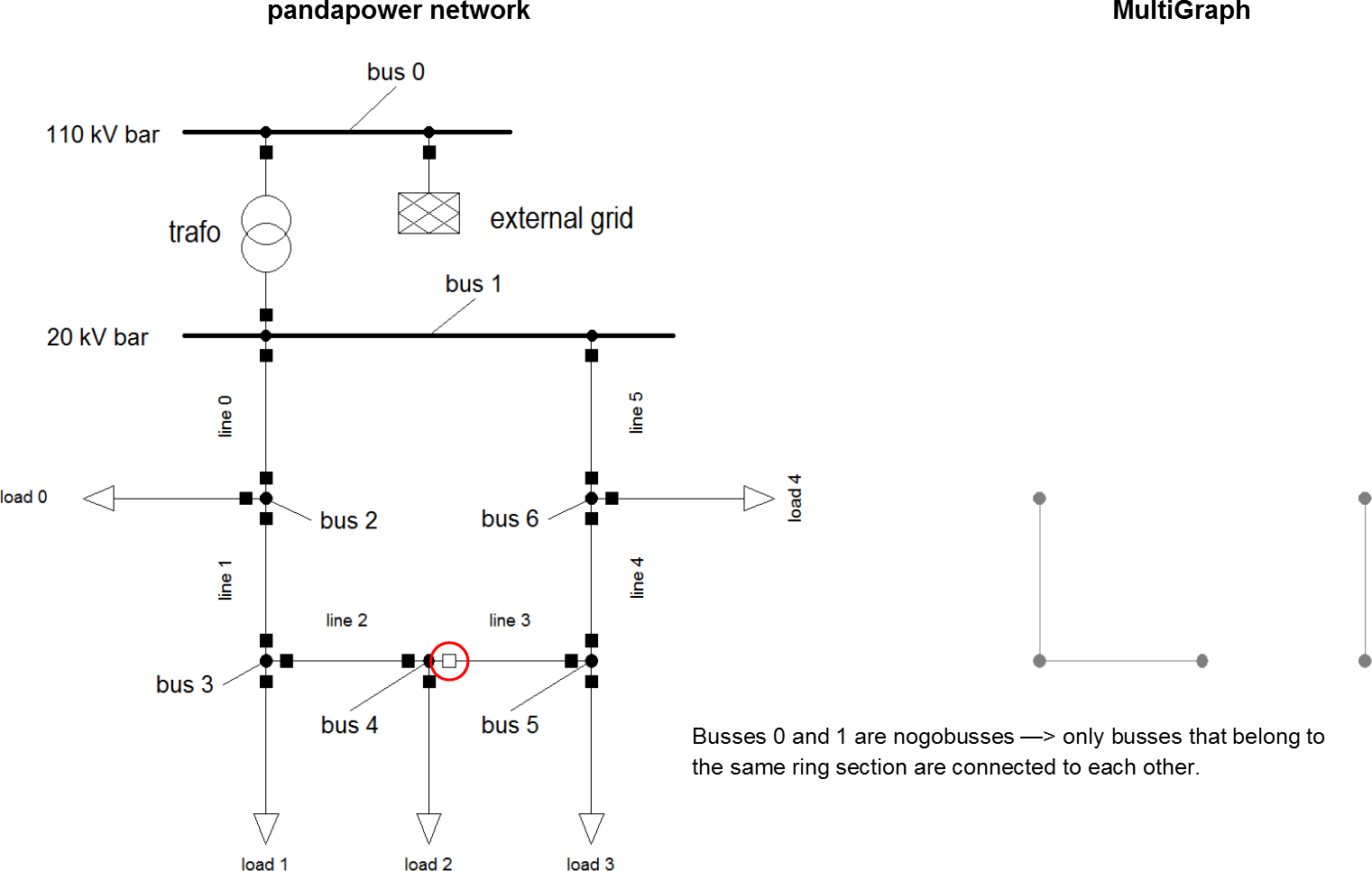```import pandapower.topology as top

mg_ring = top.create_nxgraph(net, respect_switches = False, nogobuses = [0,1])
cc_ring = top.connected_components(mg_ring)
```
```In      : next(cc_ring)
Out     : {2, 3, 4, 5, 6}
```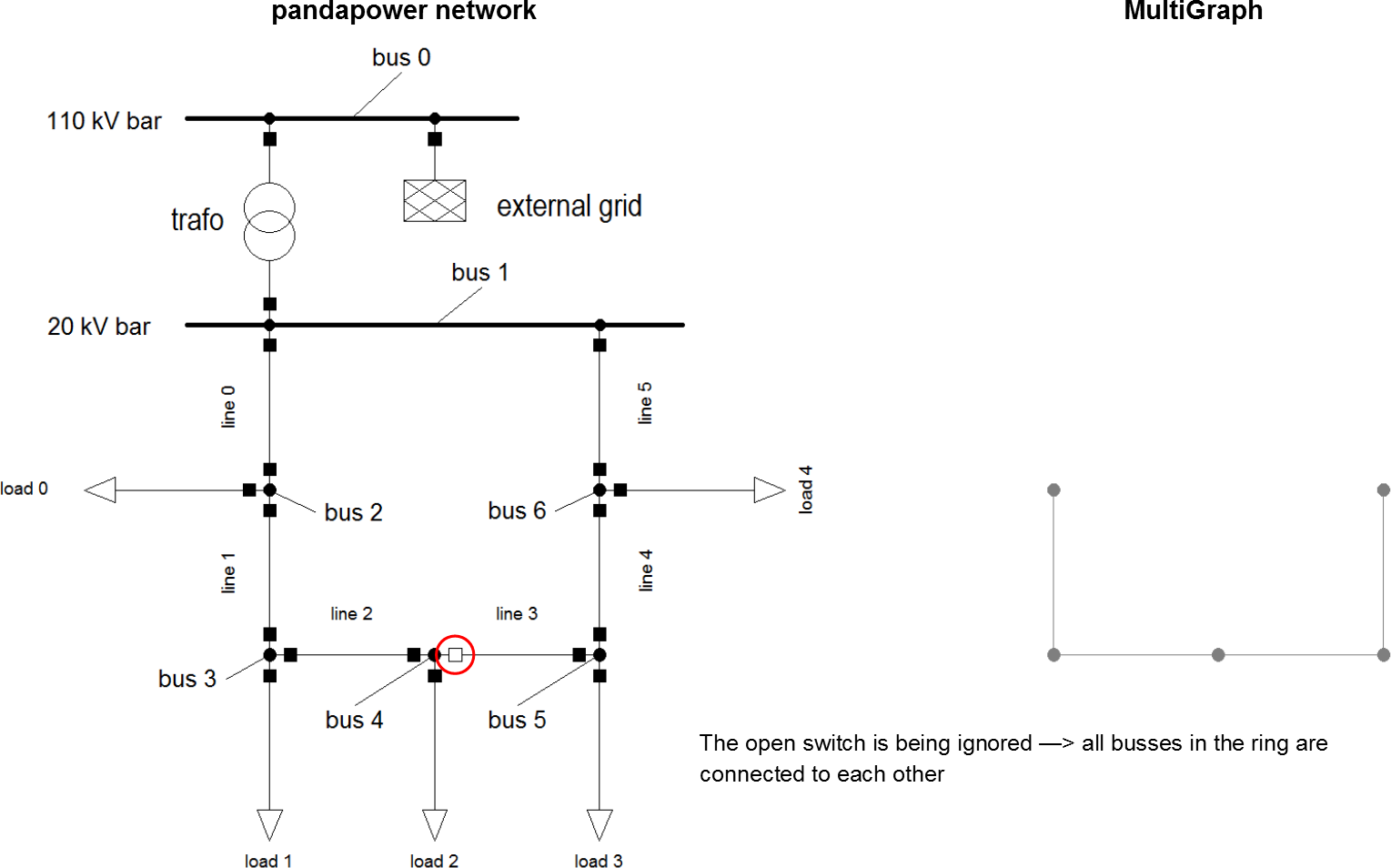## Find stubs

determine_stubs lets you identify buses and lines that are stubs. Open switches are being ignored. Busses that you want to exclude should be defined as roots. Ext_grid buses are roots by default.

This is a small extension for the example network:

```pp.create_bus(net, name = "bus 7", vn_kv = 20, type = 'b')
pp.create_bus(net, name = "bus 8", vn_kv = 20, type = 'b')

pp.create_line(net, name = "line 6", from_bus = 6, to_bus = 7, length_km = 1, std_type = "NAYY 4x150 SE")
pp.create_line(net, name = "line 7", from_bus = 7, to_bus = 8, length_km = 1, std_type = "NAYY 4x150 SE")

pp.create_load(net, 7, p_mw = 1, q_mvar = 0.2, name = "load 5")
pp.create_load(net, 8, p_mw = 1, q_mvar = 0.2, name = "load 6")
```
```import pandapower.topology as top
top.determine_stubs(net, roots = [0,1])
```
```In: net.bus

Out:
name  vn_kv type  zone  in_service  on_stub
0  110 kV bar  110.0    b  None        True    False
1   20 kV bar   20.0    b  None        True    False
2       bus 2   20.0    b  None        True    False
3       bus 3   20.0    b  None        True    False
4       bus 4   20.0    b  None        True    False
5       bus 5   20.0    b  None        True    False
6       bus 6   20.0    b  None        True    False
7       bus 7   20.0    b  None        True     True
8       bus 8   20.0    b  None        True     True

In: net.line

Out:
name       std_type  from_bus  to_bus  length_km  r_ohm_per_km    x_ohm_per_km  c_nf_per_km  g_us_per_km  max_i_ka   df  parallel type   in_service  is_stub
0  line 0  NAYY 4x150 SE         1       2        1.0         0.208           0.08        261.0          0.0      0.27  1.0         1   cs         True    False
1  line 1  NAYY 4x150 SE         2       3        1.0         0.208           0.08        261.0          0.0      0.27  1.0         1   cs         True    False
2  line 2  NAYY 4x150 SE         3       4        1.0         0.208           0.08        261.0          0.0      0.27  1.0         1   cs         True    False
3  line 3  NAYY 4x150 SE         4       5        1.0         0.208           0.08        261.0          0.0      0.27  1.0         1   cs         True    False
4  line 4  NAYY 4x150 SE         5       6        1.0         0.208           0.08        261.0          0.0      0.27  1.0         1   cs         True    False
5  line 5  NAYY 4x150 SE         6       1        1.0         0.208           0.08        261.0          0.0      0.27  1.0         1   cs         True    False
6  line 6  NAYY 4x150 SE         6       7        1.0         0.208           0.08        261.0          0.0      0.27  1.0         1   cs         True    False
7  line 7  NAYY 4x150 SE         7       8        1.0         0.208           0.08        261.0          0.0      0.27  1.0         1   cs         True    False
```